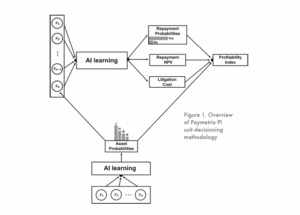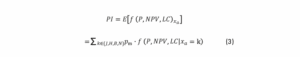This is the first in a series of posts focused on how advanced data analytics, artificial intelligence and machine learning are redefining the collections process.

Today we launch a two-part series about optimizing collections using AI.

SUIT-DECISIONING WITH PAYMETRIX PROFITABILITY INDEX (PI)

RDS has built AI-based suit-decisioning tools using our rich data to identify the most profitable accounts and prioritize the litigation process by focusing on those accounts.

Suit-decisioning is mathematically formulated as a prediction task aimed at estimating expected profitability of accounts and maximizing litigation by scoring accounts based on the estimated profitability.

RDS analyzes numerous input variables for this purpose; among these variables, Asset has been shown as one of the most significant variables. In fact, Asset significantly helps gauge the ability of debt repayment by looking into the consumer’s home and job status. Different Asset scenarios are available with respect to the consumer’s home and job status.

• If the consumer is a home owner and already employed, the Asset variable takes the value of Both (B).
• In case the consumer is only employed but renting his/her house, the asset variable is
identified as Job (J).
• For the scenario that the consumer is not employed but is a home owner, the Asset is defined
as Home (H).
• Finally, in case that consumer is not employed and is a renter, the Asset variable indicates
None (N).

Intuitively, consumers with a “Both” asset are more likely able to pay back their debts than consumers with a “None” asset. Statistical analysis on historical suit-decisioning events has also verified the predictive importance of Asset.

ASSET PREDICTION MODEL

Although Asset is a significant variable in our prediction task, verifying information on the home and job status of consumers is a timely and very expensive process (it can take up to 90 to 120 days). Some creditors may want to avoid this costly expense and make a decision quickly on their accounts, so waiting for verification of consumer asset information might not be the best option. Hence, RDS has also created an ASSET PREDICTION MODEL to accelerate suit-decisioning by predicting a consumer’s Asset. The predicted Asset will then be used instead of an actual Asset in the original model.

The overview of our suit-decisioning methodology with predicted Asset is shown in Figure 1.Our methodology uses multiple input ’s (∀ = 1 ,2 … , ) variables to predict

• Repayment probabilities
• Repayment net present value (NPV), and
• Litigation cost.

There are three output variables.

1. Repayment probability (P) measures how likely the consumers will repay the debt; thus, it is a probability value between 0 (0% chance of repayment) and 1 (100% chance of repayment).
2. Repayment NPV (NPV) measures the repayment amount in the present value.
3. Finally, litigation cost (LC) represents the expenses associated with the litigation processes such as court cost, regional costs, etc.

Our methodology adopts a multi-task learning regime, which includes the classification task of repayment probabilities and regression tasks related to repayment NPV and litigation costs.

The upper part of Figure 1 represents our suit-decisioning method assuming consumer Asset information (denoted by) is known. Given this assumption, the predicted outputs will then be used to estimate the profitability Index (PI):Ifis not known and there is a motivation to run suit-decisioning without actual information on Asset (i.e. quick and cost-effective analysis) the bottom part of Figure1 applies. Asset prediction also uses multiple inputs ’s (∀ = 1 ,2 … , ) to determine the probability of a consumer having Asset, which is designed as a multi-class classification problem with 4 classes (H, J, B, and N). The output of Asset prediction is shown as below:Due to the above probabilistic outputs, the Asset probabilities will be incorporated into the final PI analysis. In other words, we run the suit-decisioning methodology for all 4 Asset scenarios and then weight the resulted PIs with the corresponding Asset probabilities. This can be represented as an expectation of PI overas shown in the following:The proposed methodology is designed to be flexible based on the client’s goal. If the goal is more accurate suit-decisioning, which requires incurring cost and time to gain Asset information, Eq. (1) will be used, but if the goal is a quick (less than 24 hours) and cost-effective suit-decisioning then Eq. (3) will be applied (which will be less accurate).

In the next post we look at an actual banking case study where we apply the suit-decisoning model above.